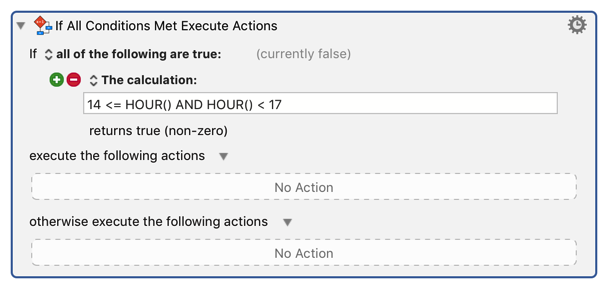# Time-based If/Then

About what you would think from the title. I want to find a way to use the current time as a conditional to feed into an if/then statement. For example: if time is between 14:00 and 17:00 do action X, if time is not between 14:00 and 17:00 do action Y.

The calculation condition, like pretty much all numeric text fields takes calculations. And you can use the HOUR() and MINUTE() functions.1 Like

This works for hours, but how do I input minutes into the time as well?

Thank you!

Generally I would just do:

1430 <= HOUR() * 100 + MINUTE() AND HOUR() * 100 + MINUTE() < 1730